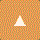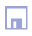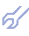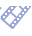In this algorithm clusterization of input data sample, optimal after balance of clusterization criterion, is found by rationally organised sorting-out procedure. It finds optimal clusterizations of input data sample among all possible clusterizations. Adequateness Law and black box concept Almost all objects of recognition and control in economy, ecology, biology and medicine are undeterministic or fuzzy. They can be represented by deterministic (robust) part and additional black boxes acting on each output of object. The only information about these boxes is that they have limited values of output variables which are similar to the corresponding states of object. According to W.R.Ashby work  diversity of control system is to be not smaller, than diversity of the object itself. The Law of Adequateness, given by S.Beer, establishes that for optimal control the objects are to be compensated by corresponding black boxes of the control system . For optimal pattern recognition and clustering only partial compensation is necessary. More of what we are interested in is to minimise the degree of compensation by all means to get more accurate results. The simplest presentation of black boxes outputs are the set of random figures with even distribution. Random figures can be compensated by another random figures only after smoothing or averaging. This operation is realised by interval discretization of the input data to D levels. According to Widrow theorem each level is to unite in itself almost equal number of the input data realisations (points). " Pointing Finger" clusterization algorithm The degree of black boxes mutual compensation can be easily regulated and optimized by balance of two clusterizations criterion calculation. It is obtained using two trees of clusterization construction.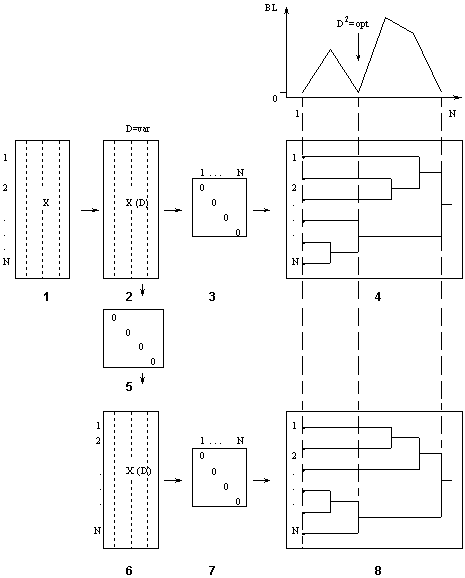There are shown:     1 - input data sample;     2 - interval discretizated sample;     3,7 - calculation of the distances between points;     4,8 - first and second hierarchical clusterization trees;     6 - interval discretizated tree, calculated with the account of analogues;     9 - calculation of number of clusters and balance criterion, which is equal BL=0 for several values of discretization levels D and several values of compensation coefficient l. The choice of the D-plane, where s=3 (two trivial clusterizations and optimal one). The realizations presented in data sample correspond to the points of multidimensional hyperspace. Each point has its nearest neighbor or first analogue. To calculate analogues the city-block measure of distance is used. Then the sample of analogues is calculated, according to weighted summation formulae: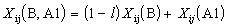, where: B - realization, given in the input data sample;           A1 - its first analogue (nearest neighbor);              l - coefficient of black boxes mutual compensation. Formulae is valid for continue-valued and interval discretizated features. For binary variables the voting procedures are developed . The hierarchical tree of clusterization is constructed for discretizated input data sample B and for the sample of analogues A1. There is proved, that the hierarchical tree construction can be considered as a procedure, which minimises the sorting-out volume: the optimal clusterization is not excluded in the result of this procedure . Then the balance of clusterizations criterion is calculated for two hierarchical trees:, where: k - number of clusters;- number of similar clusters. The pointing-out characteristic on the figure shows the change of the criterion along the steps of trees construction. Except of the tree clusterization balance criterion is to be equal to zero at the very beginning and at the end of trees construction, i.m. for clusterizations: s = 1 - every point is the separate cluster; s = N - all points are united to one cluster. Optimal clusterization we can find by means of D and l variation : D = N, N/2, N/3, ..., 2 ; l = 0, 0.05, 0.1, ... , 1. The value of noise compensation coefficient l is chosen to get single zero value of balance criterion. If the number of optimal clusterizations is cannot be reduced by increasing of l coefficient there are necessary to invite experts for final decision. It was shown that one can apply computer sorting algorithms to choosing clusterings and pattern recognition and not just to modeling [17,20]. There is no difference in general between modeling and clusterization. The difference is only in the degree of detail of the mathematical language. The language that is used is fuzzier: instead of equations, it uses cluster relationships.